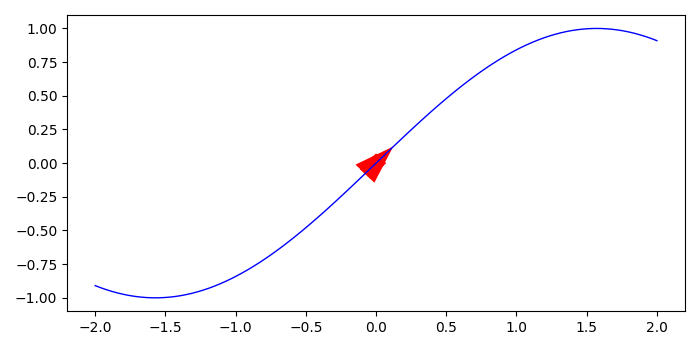# Line plot with arrows in Matplotlib

To plot with arrows in matplotlib, we can use arrow() method.

## Steps

• Create x and y data points using numpy.
• Plot x and y with color=red and linewidth = 1.
• Use arrow method to add an arrow to the axes. The first two values in the arguments are the coordinates of the arrow base and the next two values are for the length of the arrow along X and Y direction.
• To display the figure, use show() method.

## Example

from matplotlib import pyplot as plt
import numpy as np
plt.rcParams["figure.figsize"] = [7.00, 3.50]
plt.rcParams["figure.autolayout"] = True
x = np.linspace(-2, 2, 100)
y = np.sin(x)
plt.plot(x, y, c='b', lw=1)
plt.arrow(0, 0, 0.01, np.sin(0.01), shape='full', lw=10,
plt.show()